# Formula use cases

Last Updated:

Formulas in Data Explorer allow you to create reports that apply sum, ratio, and relative percentage calculations so that you can better understand behavior trends in your apps.

While the possibilities with formulas are endless, this article reviews a few common use cases for each type of function. Each use case outlines the user story of a product manager. For more step-by-step guidelines on applying formulas or building a Data Explorer report, see Data Explorer.

View the table below to understand a summary of each type of formula, then read through the specific use case examples that follow.

 Formula type Calculation Report output Sum SUM Adds usage of all events within an event group. Ratio SUM/SUM Adds usage of all events within one event group and divides that by the sum of all events within the same or another event group (can be a second event group or different measurement). Relative percentage SUMEACH/SUM Takes the total measurement (Totals, Unique Visitors, or Unique Accounts) of each event within an event group and divides that by the sum of all events within an event group.

One of the most valuable parts of formulas is that you can choose the denominator when looking at percentages for ratios and relative percentages. If you want to look at the percentage of visitors using a feature without formulas, you have to compare it to all active visitors in your subscription, not just those that have been actively engaged with a specific product area or app. Using formulas, you can limit the denominator, which gets you to the correct data that’s needed to answer your questions.

## Sum use cases

Sums help you get an aggregate understanding of how a specific set of events are used. Below are some common questions that a sum can help you answer.

### What’s the combined usage of multiple features with similar functions?

This use case requires you to add the following to your Data Explorer report:

• One event group
• Specific Features as events for group A
• Sum formula of SUM(A, Totals)### What’s the total number of page views across all of the pages within a single product area week over week?

This use case requires you to add the following to your Data Explorer report:

• One event group
• Specific Pages as events for group A
• Sum formula of SUM(A, Totals)
• Weekly as Date Range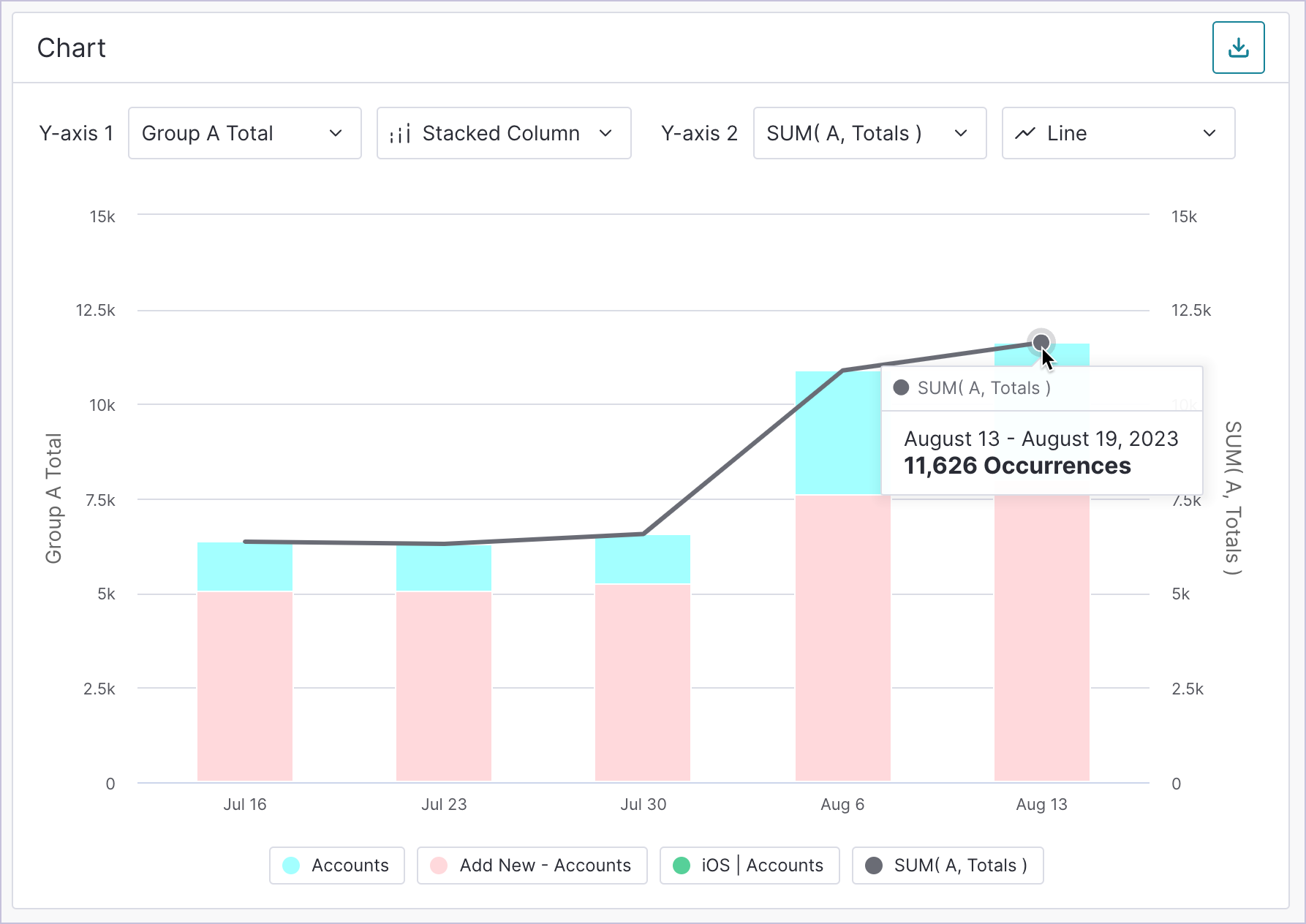### Do I have more visitors using one product area compared to another product area?

This use case requires you to add the following to your Data Explorer report:

• Two event groups
• All events within the first Product Area for group A
• All events within the second Product Area for group B
• Two Sum formulas: SUM(A, Unique Visitors) and SUM(B, Unique Visitors)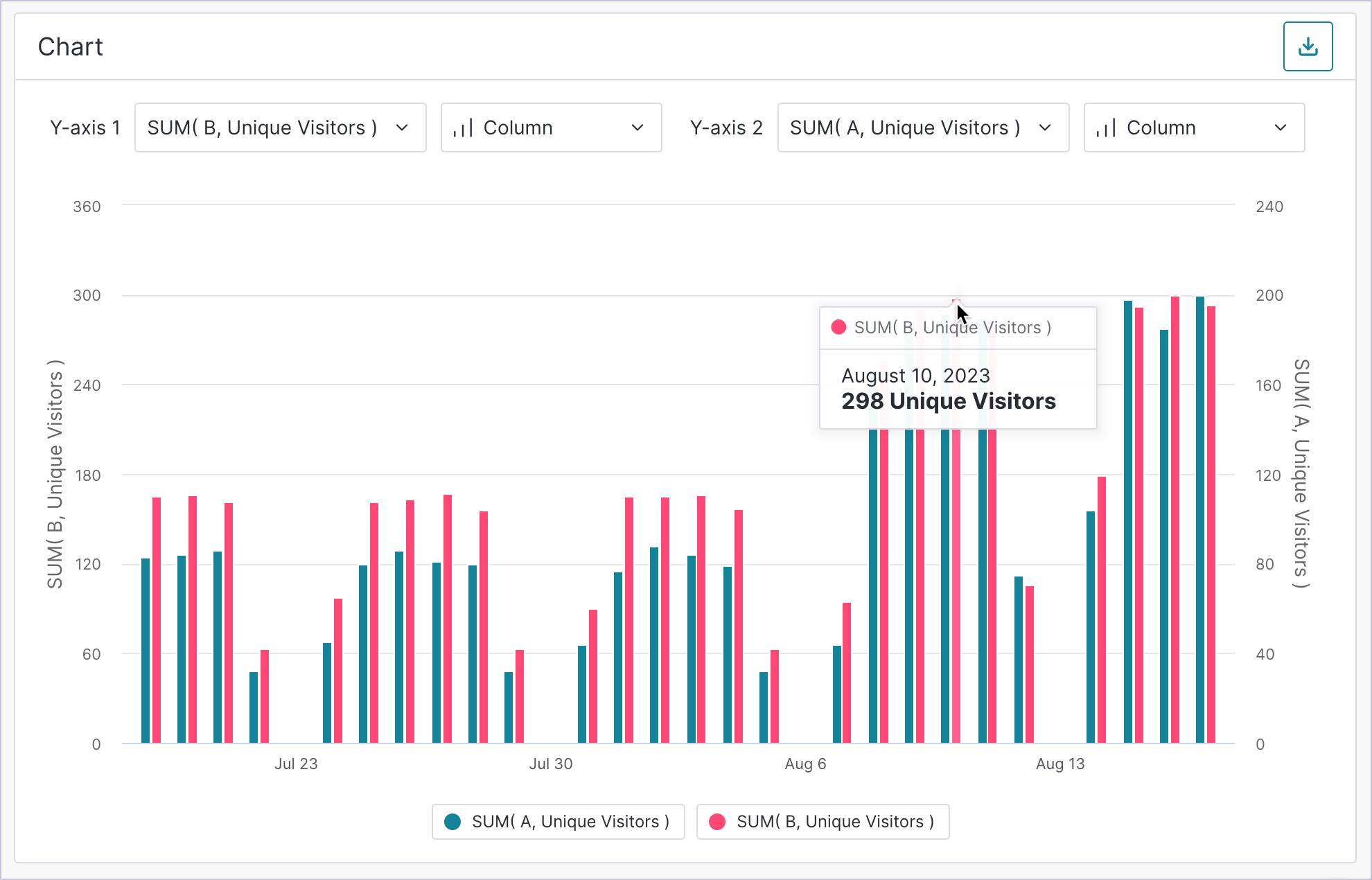## Ratio use cases

### What’s the total percentage of active mobile visitors using a specific feature in my mobile app?

This use case requires you to add the following to your Data Explorer report:

• Two event groups
• Specific Feature as event for group A
• Any Activity in specific app for group B
• Ratio formula for SUM(A, Unique Visitors) / SUM(B, Unique Visitors) as Percent### What percentage of visitors who viewed a page performed a specific task on that page?

This use case requires you to add the following to your Data Explorer report:

• Two event groups
• Specific Feature as event for group A
• Specific Page as event for group B
• Ratio formula for SUM(A, Unique Visitors) / SUM(B, Unique Visitors) as Percent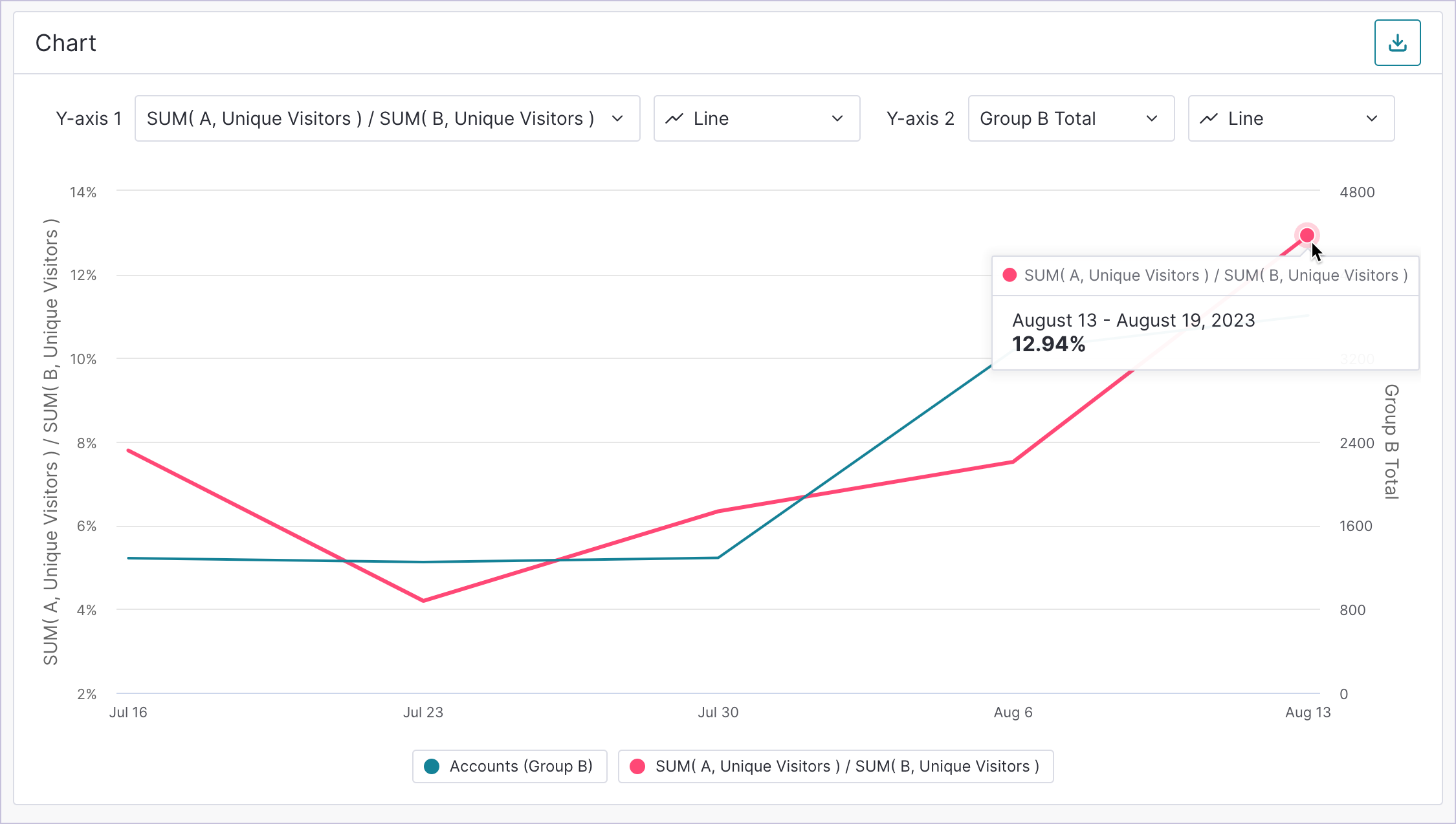## Relative percentage use cases

Relative percentages are division functions that help you understand individual event usage in relation to a larger group of events. Below are some common questions that a relative percentage can help you answer.

What’s the percentage of trial users who used our Core Events while they were in a free trial?

This use case requires you to have historical metadata enabled for your metadata field that tracks whether a visitor is currently in a free trial and to add the following to your Data Explorer report:

• Two event groups
• Core Events in specific app for group A
• Any Activity in specific app for group B
• Relative percentage formula for SUMEACH(A, Unique Visitors) / SUM(B, Unique Visitors) as Percent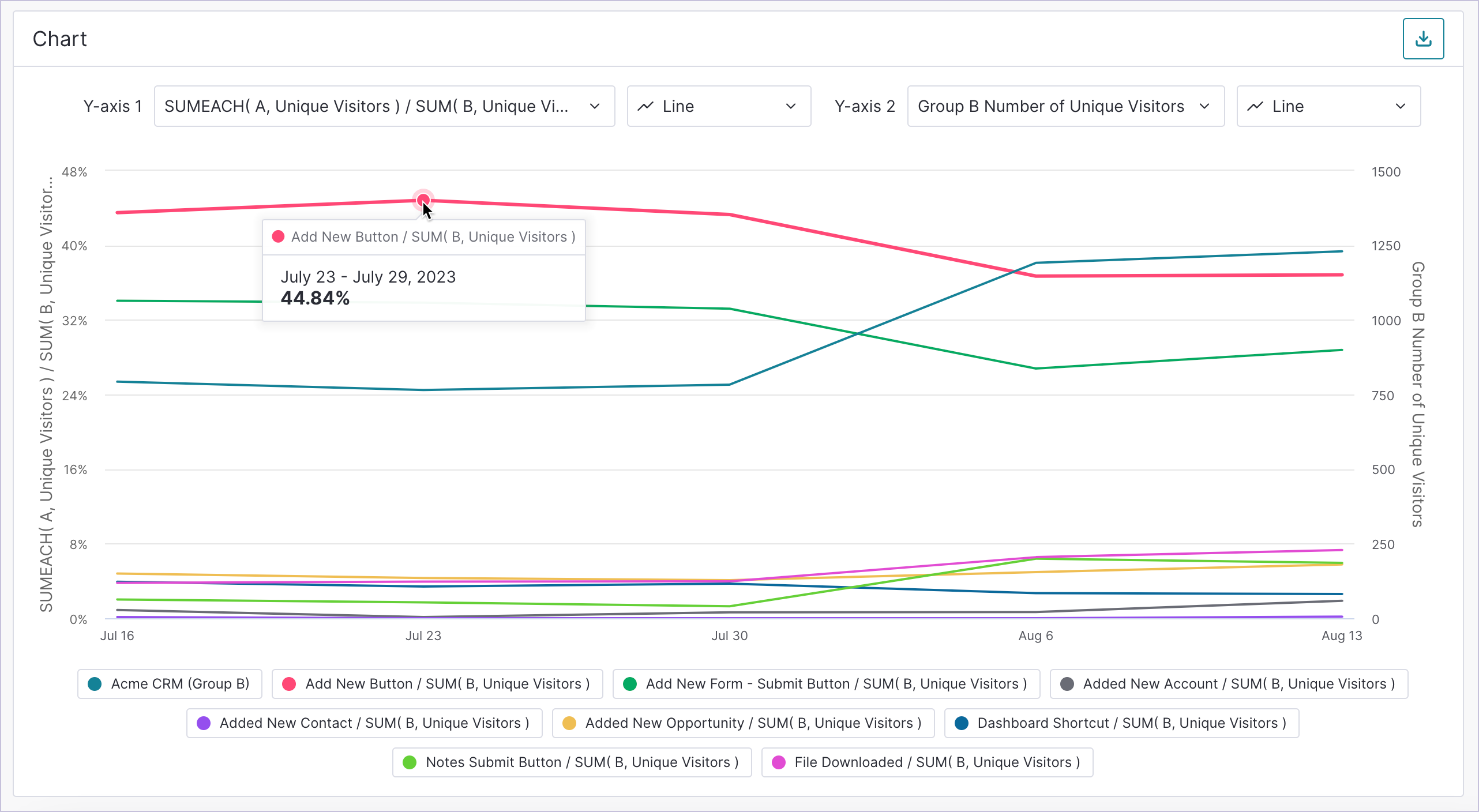### Which of my Core Events has the highest percentage of adoption compared to the total usage of all of my Core Events?

This use case requires you to add the following to your Data Explorer report:

• One event group
• Core Events selection for group A
• Relative percentage formula for SUMEACH(A, Totals) / SUM(A, Totals) as Percent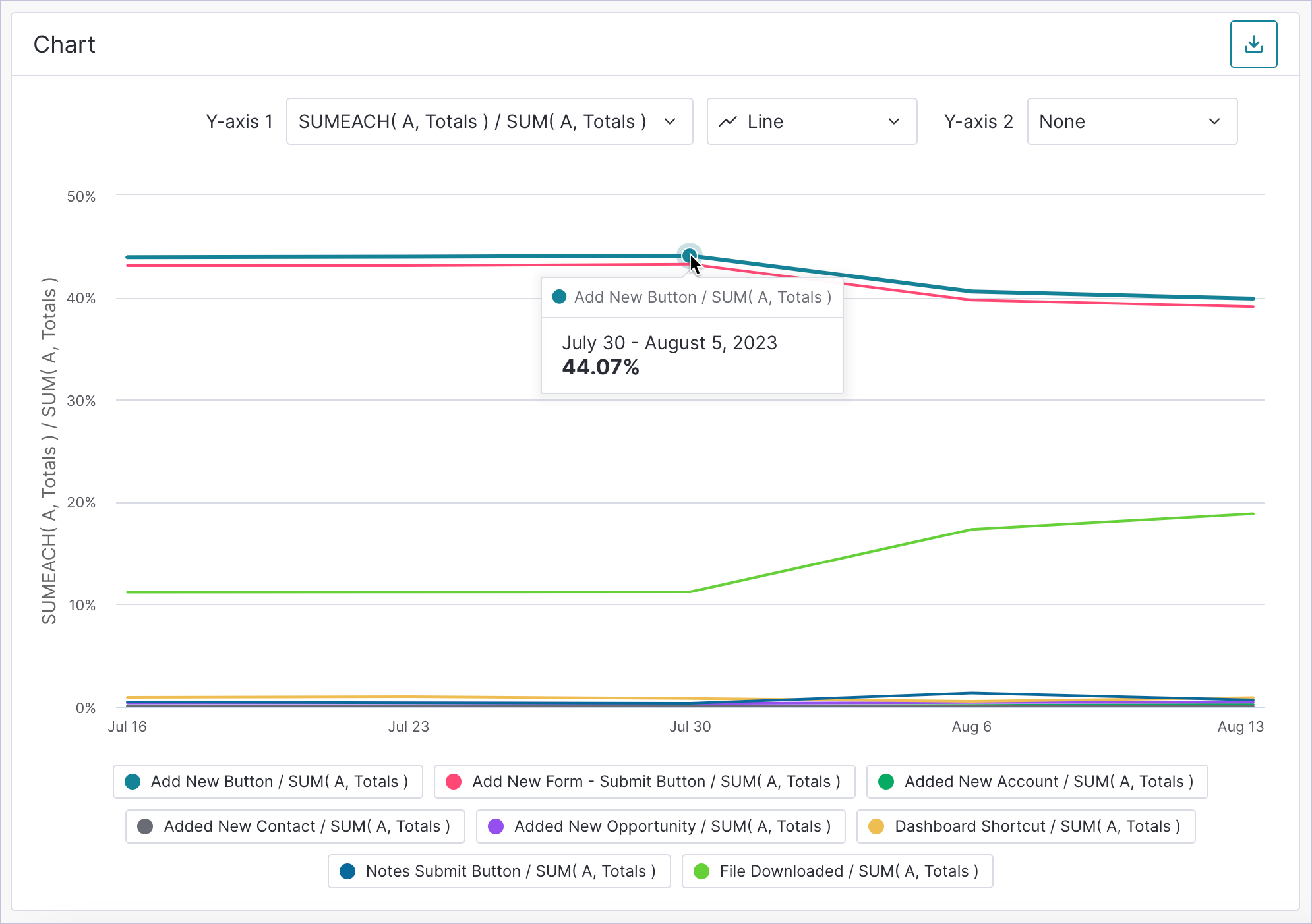## Report interpretation

No matter which formula you use for your report, the Data Explorer chart displays up to two measurements by default. Each measurement has an associated Y-axis. You can use the dropdown selector at the top of the chart to choose which measurements display on the Y-axis with the respective data.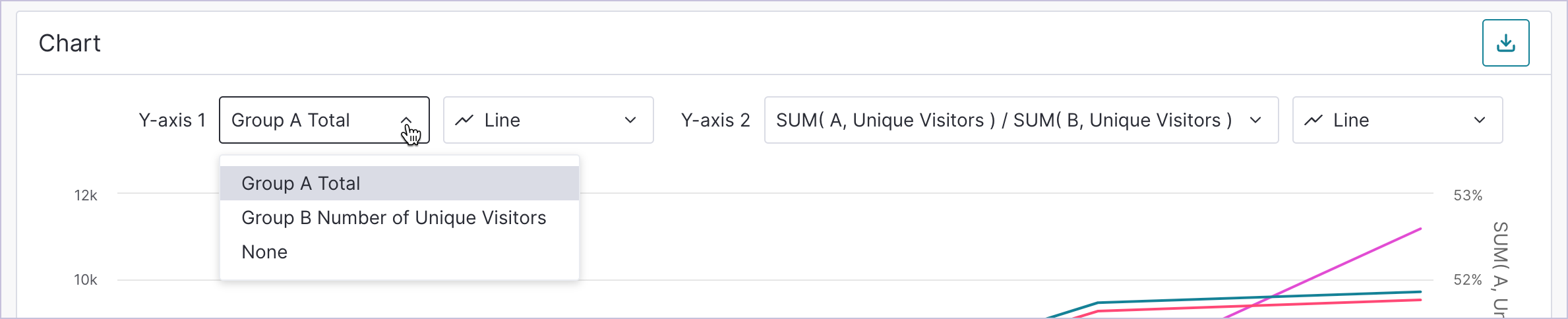Use the View (eye) icon in the breakdown table to hide or show specific data points in the chart. However, note that if your report uses more than two measurements and you choose to show additional data in the chart, some data won’t have a corresponding Y-axis.